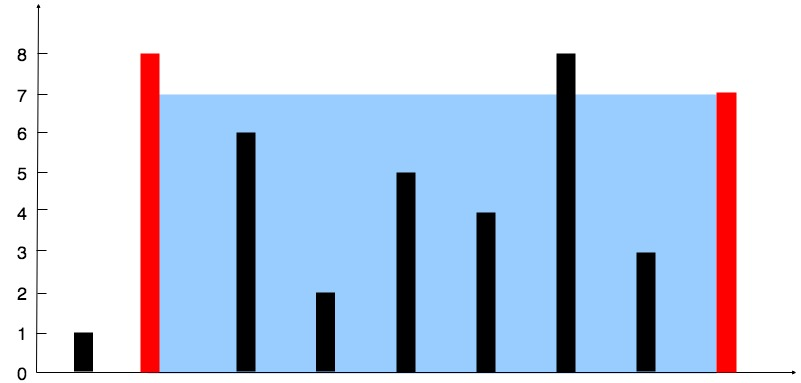2020.12.3961-芦同学

题目解答

方法一：暴力枚举法

//java
class Solution {
public int maxArea(int[] a) {

int max = 0;
for(int i =0;i<a.length-1;i++){
for(int j=i+1;j<a.length;j++){
int area = (j-i)*Math.min(a[i],a[j]);
max = Math.max(max,area)
}
}
return max;
}
}

方法二：左右边界，向中间收敛，左右夹逼

//java
class Solution {
public int maxArea(int[] height) {
int i = 0, j = height.length - 1, res = 0;
while(i < j){
//三目运算符（条件运算符，python没有？）
//？ 的意思  ：如果？前式子正确，执行第一个，不正确直行第二个
res = height[i] < height[j] ?
Math.max(res, (j - i) * height[i++]):
Math.max(res, (j - i) * height[j--]);
}
return res;
}
}
# python
class Solution:
def maxArea(self, height: List[int]) -> int:
i, j, res = 0, len(height) - 1, 0
while i < j:
if height[i] < height[j]:
res = max(res, height[i] * (j - i))
i += 1
else:
res = max(res, height[j] * (j - i))
j -= 1
return res

评论 抢沙发觉得文章有用就打赏一下文章作者

支付宝扫一扫打赏微信扫一扫打赏Vieu3.3主题Q Q 登 录